# ML Aggarwal Solutions Class 10 Maths Chapter 16 Constructions

EXERCISE 16.1

1. Use a ruler and compass only in this question.
(i) Draw a circle, centre O and radius 4 cm.
(ii) Mark a point P such that OP = 7 cm.
Construct the two tangents to the circle from P. Measure and record the length of one of the tangents.
Solution:
Allow the student to visualize the construction process.
Steps to construct:
Step 1: Draw a circle with center O and radius 4cm and mark that point as A.
Step 2: Take a point P such that OP = 7cm.
Step 3: Bisect OB at M.
Step 4: With center M and diameter OP, draw another circle intersecting the given circle at A and B.
Step 5: Join PA and PB. Hence PA and PB are pair of tangents to the circle.
Step 6: On measuring PA, it is equal to 5.5cm.2. Draw a line AB = 6 cm. Construct a circle with AB as diameter. Mark a point P at a distance of 5 cm from the mid-point of AB. Construct two tangents from P to the circle with AB as diameter. Measure the length of each tangent?
Solution:
Allow the student to visualize the construction process.
Steps to construct:
Step 1: Draw a line segment AB = 6cm.
Step 2: Draw its perpendicular bisector bisecting it at point O.
Step 3: With center O and radius OB, draw a circle.
Step 4: Extend AB to point P such that OP = 5cm.
Step 5: Draw its perpendicular bisector intersecting it at point M.
Step 6: With center M and radius OM, draw a circle which intersects the given circle at T and S.
Step 7: Join OT,OS,PT and PS. Hence, PT and PS are the required tangents to the given circle.
Step 8: On measuring each tangent is 4cm long. PT = PS = 4cm.3. Construct a tangent to a circle of radius 4cm from a point on the concentric circle of radius 6 cm and measure its length. Also, verify the measurement by actual calculation.
Solution:
Allow the student to visualize the construction process.
Steps to construct:
Step 1: Mark a point O.
Step 2: With center O and radius 4cm and 6cm, draw two concentric circles.
Step 3: Join OA and mark its mid-point as M.
Step 4: With center M and radius MA, draw another circle which intersects the first circle at P and Q.
Step 5: Join AP and AQ. Hence, AP and AQ are the required tangents to the first circle from point A.4. Draw a circle of radius 3 cm. Take two points P and Q on one of its extended diameter each at a distance of 7 cm from its centre. Draw tangents to the circle from these two points P and Q.
Solution:
Allow the student to visualize the construction process.
Steps to construct:
Step 1: Consider a point O on a line, with center O, and radius 3cm, draw a circle.
Step 2: Extend its diameters on both sides and cut off OP = OQ = 7cm.
Step 3: Mark the mid-points of OP and OQ as M and N respectively.
Step 4: With Centers M and N and OP and OQ as diameters, draw circles which intersect the given circle at A,B and C,D respectively.
Step 5: Join PA,PB,QC,QD. Hence, PA,PB and QC,QD are the required tangents.5. Draw a line segment AB of length 8 cm. Taking A as centre, draw a circle of radius 4 cm and taking B as centre, draw another circle of radius 3 cm. Construct tangents to each circle from the centre of the other circle.
Solution:
Allow the student to visualize the construction process.
Steps to construct:
Step 1: Draw a line segment AB = 8cm.
Step 2: With center as A and radius 4cm, with center as B and radius 3cm, draw circles.
Step 3: Draw the third circle AB as diameter which intersects the given two circles at C and D,P and Q respectively.
Step 4: Join AC,AD,BP,BQ. Hence, AC and AD,BP and BQ are the required tangents.EXERCISE 16.2

1. Draw an equilateral triangle of side 4 cm. Draw its circumcircle.
Solution:
Allow the student to visualize the construction process.
Steps to construct:
Step 1: Draw a line segment BC = 4cm.
Step 2: With centers B and C, draw two arcs of radius 4cm which intersects each other at point A.
Step 3: Join AB and AC.
Step 4: Draw the right bisector of BC and AC intersecting each other at point O.
Step 5: Join OA,OB and OC.
Step 6: With center as O, and radius equal to OB or OC or OA, draw a circle which passes through points A, B and C.
Hence the required circumcircle of triangle ABC is given below.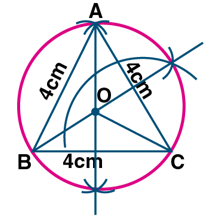2. Using a ruler and a pair of compasses only, construct:
(i) A triangle ABC given AB = 4 cm,BC = 6 cm and ∠ABC = 90°.
(ii) A circle which passes through the points A,B and C and mark its centre as O.
Solution:
Allow the student to visualize the construction process.
Steps to construct:
Step 1: Draw a line segment AB = 4cm.
Step 2: At point B, draw a ray BX making an angle of 90° and cut off BC = 6cm.
Step 3: Join AC.
Step 4: Draw the perpendicular bisectors of sides AB and AC intersecting each other at point O.
Step 5: With center as O, and radius equal to OB or OA or OC, draw a circle which passes through points A,B,C.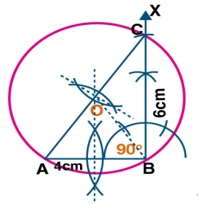3. Construct a triangle with sides 3 cm,4 cm and 5 cm. Draw its circumcircle and measure its radius.
Solution:
Allow the student to visualize the construction process.
Steps to construct:
Step 1: Draw a line segment BC = 4cm.
Step 2: With Center as B and radius 3cm, with center as C and radius 5cm draw two arcs which intersect each other at point A.
Step 3: Join AB and AC.
Step 4: Draw the perpendicular bisector of sides BC and AC which intersects each other at point O.
Step 5: Join OB.
Step 6: With center as O and radius OB, draw a circle which pass through A,B,C.
Step 7: On measuring the radius OB = 2.5cm.4. Using a ruler and compasses only:
(i) Construe a triangle ABC with the following data:
Base AB = 6 cm,AC = 5.2 cm and ∠CAB = 60°.
(ii) In the same diagram, draw a circle which passes through the points A,B and C. and mark its centre O.
Solution:
Allow the student to visualize the construction process.
Steps to construct:
Step 1: Draw a line segment AB = 6cm.
Step 2: At point A, draw a ray making an angle of 60°.
Step 3: With B as the center and radius 5.2cm, draw an arc which intersects the ray at C.
Step 4: Join BC.
Step 5: Draw the perpendicular bisector of sides AB and BC which intersects each other at point O.
Step 6: With center as O and radius OA, draw a circle which touches through the points A,B,C.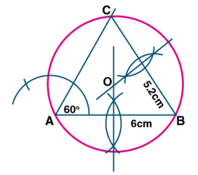5. Using ruler and compasses only, draw an equilateral triangle of side 5 cm and draw its inscribed circle. Measure the radius of the circle.
Solution:
Allow the student to visualize the construction process.
Steps to construct:
Step 1: Draw a line segment BC = 5cm.
Step 2: With Center as B and radius 5cm, with center as C and radius 5cm draw two arcs which intersect each other at point A.
Step 3: Join AB and AC.
Step 4: Draw the angle bisector of angles B and C which intersects each other at point I.
Step 5: From I, draw a perpendicular ID on BC.
Step 6: With center as I and radius ID, draw a circle which touches the sides of the triangle internally.
Step 7: On measuring the radius ID = 1.5cm (approx).6. (i) Conduct a triangle ABC with BC = 6.4 cm,CA = 5.8 cm and ∠ ABC = 60°. Draw its incircle. Measure and record the radius of the incircle.
(ii) Construct a ∆ABC with BC = 6.5 cm,AB = 5.5 cm,AC = 5 cm. Construct the incircle of the triangle. Measure and record the radius of the incircle.
Solution:
Allow the student to visualize the construction process.
Steps to construct:
Step 1: Draw a line segment BC = 6.4cm.
Step 2: Construct an angle of 60° at point B.
Step 3: With C as center and radius CA = 5.8cm, draw an arc cutting BD at A.
Step 4: Join AC.
Step 5: Draw the angle bisector of angle B and angle C which intersect each other at point O.
Step 6: Draw OE perpendicular to BC, intersecting BC at point E.
Step 7: With O as the center and OE as the radius draw the required incircle.
Step 8: On measuring the radius OE = 1.5cm.Allow the student to visualize the construction process.
Step 1: Draw a line segment BC = 6.5cm.
Step 2: With B as center and C as center draw arcs AB = 5.5cm and AC = 5cm.
Step 3: Join AB and AC.
Step 4: Draw the angle bisectors of B and C. The bisectors meet at point O.
Step 5: With O as the center. Draw an incircle which touches all the sides of the triangle ABC.
Step 6: From point O draw a perpendicular to side BC which cuts at point N.
Step 7: On measuring the radius ON = 1.5cm.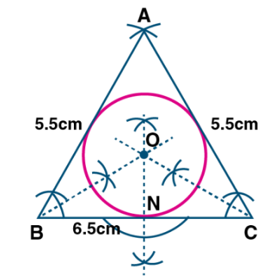7. The bisectors of angles A and B of a scalene triangle ABC meet at O.
(i) What is the point O called?
(ii) OR and OQ is drawn a perpendicular to AB and CA respectively. What is the relation between OR and OQ?
(iii) What is the relation between ∠ACO and ∠BCO?
Solution:
(i) The point O where the angle bisectors meet is called the incenter of the triangle.
(ii) The perpendicular drawn from point O to AB and CA are equal. i.e., OR and OQ.
(iii) ∠ACO = ∠BCO.OC will bisect the ∠C.8. Using ruler and compasses only, construct a triangle ABC in which BC = 4 cm,∠ACB = 45° and the perpendicular from A on BC is 2.5 cm. Draw the circumcircle of triangle ABC and measure its radius.
Solution:
Allow the student to visualize the construction process.
Steps to construct:
Step 1: Draw a line segment BC = 4cm.
Step 2: At point B, draw a perpendicular and cut off BE = 2.5cm.
Step 3: From, E, draw a line EF parallel to BC.
Step 4: From point C, draw a ray making an angle 45° which intersects EF at point A.
Step 5: Join AB.
Step 6: Draw the perpendicular bisectors of sides BC and AC intersecting each other at point O.
Step 7: Join OB,OC and OA.
Step 8: With O as the center and radius OB or OC or OA draw a circle which passes through points A,B,C.
Step 9: On measuring the radius OB = 2cm.9. Construct a regular hexagon of side 4 cm. Construct a circle circumscribing the hexagon.
Solution:
Allow the student to visualize the construction process.
Steps to construct:
Step 1: Draw a line segment AB = 4cm.
Step 2: At points A and B draw rays making on angle of 120° each and cut off AF = BC = 4cm.
Step 3: At point C and F draw rays making on angle of 120° each and cut off FE = CD = 4cm.
Step 4: Join ED. The required ABCDEF hexagon is formed.
Step 5: Draw perpendicular bisectors of sides AB and BC intersecting each other at point O.
Step 6: With O as the center and radius equal OA or OB draw a circle which passes through the vertices of the hexagon.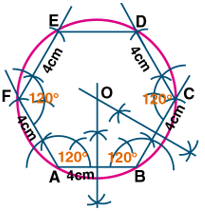10. Draw a regular hexagon of side 4 cm and construct its incircle.
Solution:
Allow the student to visualize the construction process.
Steps to construct:
Step 1: Draw a regular hexagon of sides 4cm.
Step 2: Draw the angle bisector of A and B. which intersects each other at point O.
Step 3: Draw OL perpendicular to AB.
Step 4: With O as the center and radius OB, draw a circle which touches the sides of the hexagon.
Step 5: Use a straightedge and pencil to construct the sides of regular hexagon ABCDEF.